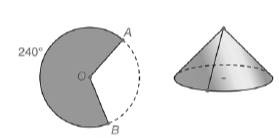Chapter 9.3, Problem 48EElementary Geometry For College St...

7th Edition
Alexander + 2 others
ISBN: 9781337614085

Solutions

Chapter
SectionElementary Geometry For College St...

7th Edition
Alexander + 2 others
ISBN: 9781337614085
Textbook Problem

When radii O A ¯ and O B ¯ are placed so that they coincide, a 240 ∘ sector of a circle is sealed to form a right circular cone. If the length of the radius of the circle is 6.4 cm, what is the lateral area of the cone that is formed? Use a calculator and round the answer to the nearest tenth of a square inch.To determine

To find:

The approximate lateral area of the cone that is formed.

Explanation

Given:

When radii OA and OB are placed so that they coincide, a 240 sector of a circle is sealed to form a right circular cone. The radius of the circle is 6.4 cm.

Calculation:

In a circle of radius r, the area of the sector whose arc measure is m is A=m360πr2.

We know 1 inch=2.54 cm

We have r=6.4 cm

r=6.42.54 in2.52 in

We have m=240,r=2

Still sussing out bartleby?

Check out a sample textbook solution.

See a sample solution

The Solution to Your Study Problems

Bartleby provides explanations to thousands of textbook problems written by our experts, many with advanced degrees!

Get Started

Find more solutions based on key concepts